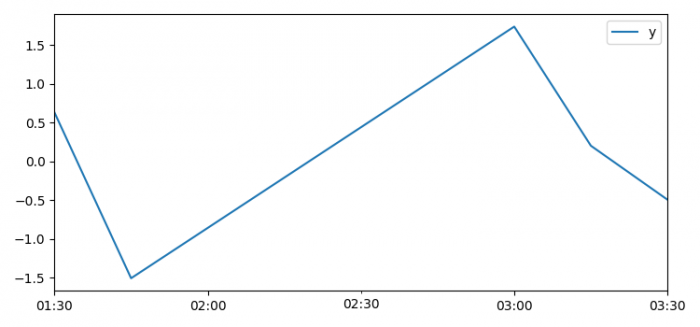# How to handle times with a time zone in Matplotlib?

To handle times with a time zone in Matplotlib, we can take the following steps −

• Set the figure size and adjust the padding between and around the subplots.
• Create a dataframe, i.e., two-dimensional, size-mutable, potentially heterogeneous tabular data.
• To handle times with a time zone, use pytz library that brings the Olson tz database into Python. This library allows accurate and cross-platform timezone calculations.
• Plot the dataframe using plot() method.
• To display the figure, use show() method.

## Example

import pandas as pd
import numpy as np
from matplotlib import pyplot as plt
import pytz

plt.rcParams["figure.figsize"] = [7.50, 3.50]
plt.rcParams["figure.autolayout"] = True

df = pd.DataFrame(
dict(y=np.random.normal(size=5)),
index=pd.date_range(
start='2018-03-11 01:30',
freq='15min',
periods=5,
tz=pytz.timezone('US/Eastern')))
df.plot()

plt.show()

## Output